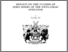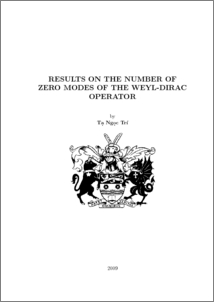# Results on the number of zero modes of the Weyl-Dirac operator

Ta, Tri (2009) Results on the number of zero modes of the Weyl-Dirac operator. PhD thesis, UNSPECIFIED.Preview
PDF (ThesisV2.pdf)
ThesisV2.pdf

## Abstract

For a given magnetic potential A one can define the Weyl-Dirac operator σ·(−i∇−A) on R 3 . An L 2 eigenfunction of σ · (−i∇ − A) corresponding to 0 is called a zero mode. In this thesis we will be concerned with the zero mode problem for the WeylDirac operator and some related problems. The main results are (i) upper bounds for the number of zero modes of the Weyl-Dirac operator in three dimensions when scaling a given magnetic field. A similar version for the Dirac operator in two dimensions is also obtained. There are also related results to estimate the number of zero modes of the massless Dirac operator, and the dimension of the eigenspaces at threshold energies for the Dirac operator with positive mass. (ii) construction of Dirac operators on the unit ball S 2 of R 3 as well as the determination of their spectrum in case of “constant” magnetic fields. We also show another proof for the Aharonov-Casher theorem for S 2 based on results about spectral properties of Dirac operators that we have obtained. (iii) a formula giving the number of zero modes of the Weyl-Dirac operator for a special magnetic field, which is the result of pullbacks from the “constant” volume form of S 2 . We also obtain a lower bound for the number of zero modes for the Weyl-Dirac operator corresponding to certain scaled magnetic fields; the magnetic fields are parallel to fibres of the Hopf fibration (pulled-back to R 3 using inverse stereographic projection).

Item Type:
Thesis (PhD)
Uncontrolled Keywords:
/dk/atira/pure/researchoutput/libraryofcongress/qa
Subjects:
ID Code:
30804
Deposited By:
Deposited On:
07 Dec 2009 09:31
Refereed?:
No
Published?:
Published